# 一、直方图

语法：
- images:原图像格式为uint8或float32，当传入函数时需要用[]括起来使用；
- channels: 同样用中括号括起来，告诉程序统计图像的直方图。如果是灰度图，就为,如果是彩色图像，传入参数为,对应着BGR
- histSize:BIN,分组范围，直方图的横坐标，也用中括号[]；
- ranges:像素值范围，一般常用[0,256]

def cv_show(img):
cv2.imshow("img",img)
cv2.waitKey(0)
cv2.destroyWindow('all')

'''
plt.hist(img.ravel(),256,[0,256],facecolor ='black')
- img.ravel()–把多维数组转化成一维数组，因为hist函数只支持一维的数组（数组下标为横坐标，值为纵坐标）
- 256 表示横坐标的最大值为256，有256条柱
- [0,256]表示数据显示范围 。PS：横坐标超过256的数据也归到256处
- facecolor 表示柱状图的颜色
'''
plt.hist(img.ravel(),256);
plt.show()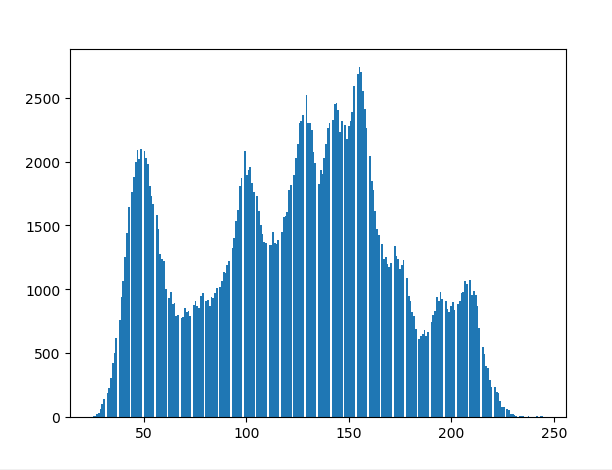#彩色图直方图分布
color = ['b','g','r']
for i ,col in enumerate(color):
histr = cv2.calcHist([img],[i],None,,[0,256])
plt.plot(histr,color=col)
plt.xlim([0,256]) #设置plt画板x轴的上下限，就是x轴所显示的长度。
plt.show()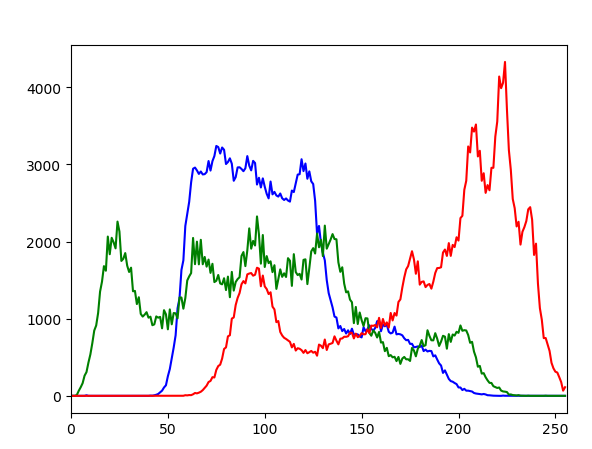二、掩模 mask操作

#对比 整个图像的 直方图 和 带有掩码部分的直方图

hist_all = cv2.calcHist([img_gray],,None,,[0,256])

plt.subplot(321),plt.imshow(img,'gray')
plt.subplot(323),plt.imshow(img_gray,'gray')
plt.subplot(325),plt.plot(hist_all)
plt.xlim([0,256])
plt.show()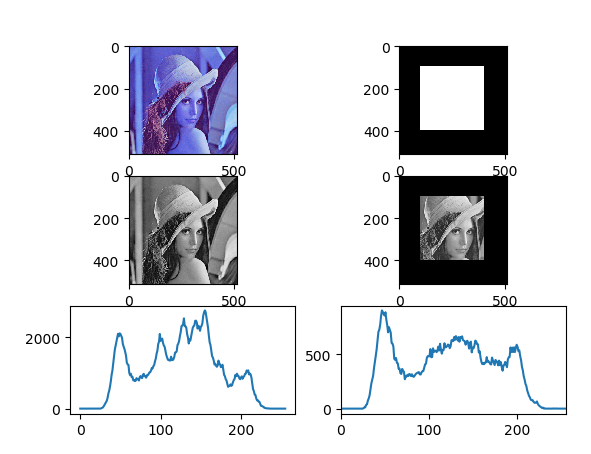## （1） 图像均衡化

是的直方图分布均匀，高瘦 变成 矮胖，但是有可能缺失细节，因为是基于全局做的均衡化。

cv2.equalizeHist(img)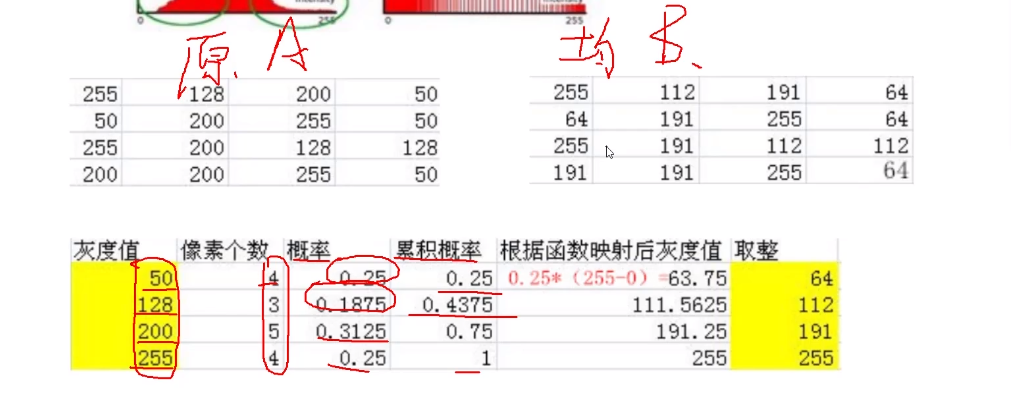# 全局图像均衡化
# 分布均匀，高瘦 变成 矮胖，但是有可能缺失细节，因为是基于全局做的均衡化

#累计概率，然后根据函数映射，取整。
#hist = cv2.calcHist([img],,None,256,[0,256])
plt.hist(img.ravel(),256);
plt.show()

# 均衡化  # 变得 矮胖
equ = cv2.equalizeHist(img)
plt.hist(equ.ravel(),256)
plt.show()

vs = np.hstack((img,equ))
cv_show(vs)   # 更亮了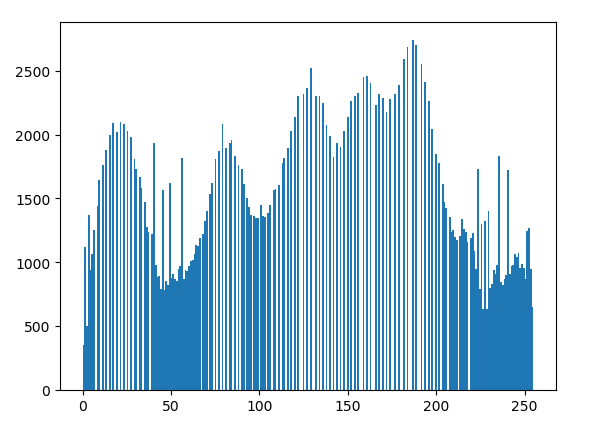二、自适应直方图均衡化

cv2.createCLAHE(clipLimit=2.0,tileGridSize=(8,8))

clipLimit 颜色对比度的阈值
titleGridSize 进行像素均衡化的网格大小，即在多少网格下进行直方图的均衡化操作

# 分块做，效果好

clahe = cv2.createCLAHE(clipLimit=2.0,tileGridSize=(8,8))# 1、颜色对比度的阈值 2、每一块大小 8*8

res_clahe = clahe.apply(img)

res = np.hstack((img,equ,res_clahe))
cv_show(res)# 二、傅里叶变换

cv2.dft() #傅里叶变换
cv2.idft()# 逆变换

#傅里叶变换的作用
- 高频：变化剧烈的灰度分量，例如边界；
- 低频：变化缓慢的灰度分量，例如一片大海；

# 滤波
- 低通滤波器：只保留低频，会使得图像模糊；
- 高通滤波器：只保留高频，会使得图像细节增强；

- openCV中主要就是cv2.dft()和cv2.idft(),输入图像需要先转换成np.float32格式。
- 得到的结果中频率为0的部分会在左上角，通常要转换到中心位置，可以通过shift变换来实现。
- cv2.dft()返回的结果是双通道的（实部和虚部），通常还需要转换成图像格式才能展示（0,255）。

 # 1、读取图像，并转换为 float32格式
img_float32 = np.float32(img)

# 2、进行傅里叶变换
dft = cv2.dft(img_float32,flags=cv2.DFT_COMPLEX_OUTPUT)#频谱图
dft_shift = np.fft.fftshift(dft) # 低频值转换到中间位置，将零频点移到频谱的中间
'''
cv2.magnitude()  #计算二维矢量的幅值
'''
# 3、得到灰度图能表示的形式，但是数值较小，映射到[0,255]之间
magnitude_spectrum = 20*np.log(cv2.magnitude(dft_shift[:,:,0],dft_shift[:,:,1]))

plt.subplot(121),plt.imshow(img)
plt.title('input image'),plt.xticks([]),plt.yticks([])
plt.subplot(122),plt.imshow(magnitude_spectrum)
plt.title('magnitude_spectrum'),plt.xticks([]),plt.yticks([])
plt.show()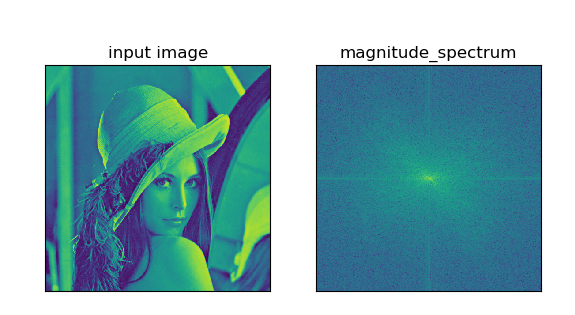## （1）低通滤波

低通滤波器：只保留低频，会使得图像模糊；

## 低通滤波器

# 1、读取图像，并转换为 float32格式
img_float32 = np.float32(img)

# 2、进行傅里叶变换
dft = cv2.dft(img_float32,flags=cv2.DFT_COMPLEX_OUTPUT)#频谱图
dft_shift = np.fft.fftshift(dft) # 低频值转换到中间位置，将零频点移到频谱的中间

rows,cols = img.shape
crow,ccol = int(rows/2),int(cols/2) # 得到中心位置

# 3、低通滤波

# idft   逆向佛里叶
f_ishift = np.fft.ifftshift(fshift)# 从中间还原位置到原来的 左上角
img_back = cv2.idft(f_ishift) # 逆向佛里叶
img_back = cv2.magnitude(img_back[:,:,0],img_back[:,:,1])

plt.subplot(121),plt.imshow(img)
plt.title('input image'),plt.xticks([]),plt.yticks([])
plt.subplot(122),plt.imshow(img_back)
plt.title('result'),plt.xticks([]),plt.yticks([])
plt.show()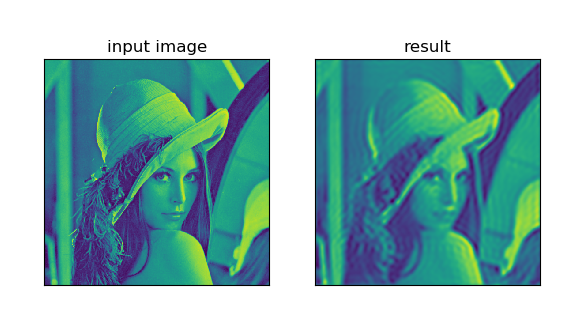## （2）高通滤波

高通滤波器：只保留高频，会使得图像细节增强；

# 1、读取图像，并转换为 float32格式
img_float32 = np.float32(img)

# 2、进行傅里叶变换
dft = cv2.dft(img_float32,flags=cv2.DFT_COMPLEX_OUTPUT)#频谱图
dft_shift = np.fft.fftshift(dft) # 低频值转换到中间位置，将零频点移到频谱的中间

rows,cols = img.shape
crow,ccol = int(rows/2),int(cols/2) # 得到中心位置

# 3、高通滤波

# idft   逆向佛里叶
f_ishift = np.fft.ifftshift(fshift)# 从中间还原位置到原来的 左上角
img_back = cv2.idft(f_ishift) # 逆向佛里叶
img_back = cv2.magnitude(img_back[:,:,0],img_back[:,:,1])

plt.subplot(121),plt.imshow(img)
plt.title('input image'),plt.xticks([]),plt.yticks([])
plt.subplot(122),plt.imshow(img_back)
plt.title('result'),plt.xticks([]),plt.yticks([])
plt.show()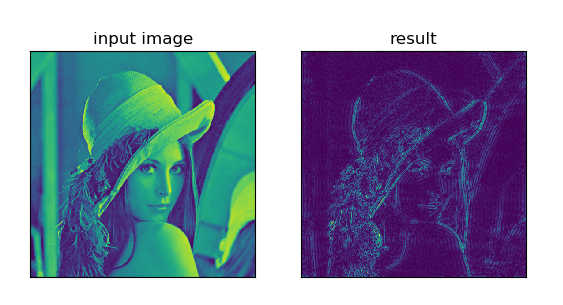### 利用POI将Excel文档转换为Html_jsudavid的博客-程序员秘密

excel文档转换为html其实和word转html相类似，优点也很明显。请参考 word转html        public static void convertExcel2Html(String excelFilePath,String htmlFilePath) throws IOException,ParserConfigurationException,Transforme

### echarts多个图形自适应的写法_is17的博客-程序员秘密

window.addEventListener("resize", () =&gt; { myChart.resize(); });

### SpringBoot报错Failed to start component [StandardEngine[Tomcat].StandardHost[localhost].TomcatEmbedded_Vove7的博客-程序员秘密

Failed to start component [StandardEngine[Tomcat].StandardHost[localhost].TomcatEmbeddedContext[]]Application startup failedorg.springframework.context.ApplicationContextException: Unable to start embedded